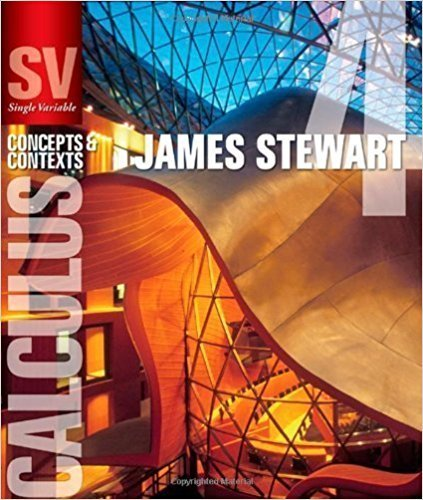×

×

# Solutions for Chapter 1.1: FUNCTIONS AND MODELS## Full solutions for Single Variable Calculus: Concepts and Contexts (Stewart's Calculus Series) | 4th Edition

ISBN: 9780495559726Solutions for Chapter 1.1: FUNCTIONS AND MODELS

Solutions for Chapter 1.1
4 5 0 430 Reviews
21
3
##### ISBN: 9780495559726

Since 74 problems in chapter 1.1: FUNCTIONS AND MODELS have been answered, more than 64531 students have viewed full step-by-step solutions from this chapter. This expansive textbook survival guide covers the following chapters and their solutions. Single Variable Calculus: Concepts and Contexts (Stewart's Calculus Series) was written by and is associated to the ISBN: 9780495559726. This textbook survival guide was created for the textbook: Single Variable Calculus: Concepts and Contexts (Stewart's Calculus Series), edition: 4. Chapter 1.1: FUNCTIONS AND MODELS includes 74 full step-by-step solutions.

Key Calculus Terms and definitions covered in this textbook
• Acceleration due to gravity

g ? 32 ft/sec2 ? 9.8 m/sec

• Anchor

See Mathematical induction.

• Closed interval

An interval that includes its endpoints

• Combinations of n objects taken r at a time

There are nCr = n! r!1n - r2! such combinations,

• Demand curve

p = g(x), where x represents demand and p represents price

• Dot product

The number found when the corresponding components of two vectors are multiplied and then summed

• Frequency

Reciprocal of the period of a sinusoid.

• Horizontal line

y = b.

• Initial side of an angle

See Angle.

• Mathematical model

A mathematical structure that approximates phenomena for the purpose of studying or predicting their behavior

• Multiplicative identity for matrices

See Identity matrix

• Number line graph of a linear inequality

The graph of the solutions of a linear inequality (in x) on a number line

• Opens upward or downward

A parabola y = ax 2 + bx + c opens upward if a > 0 and opens downward if a < 0.

• Parametric equations for a line in space

The line through P0(x 0, y0, z 0) in the direction of the nonzero vector v = <a, b, c> has parametric equations x = x 0 + at, y = y 0 + bt, z = z0 + ct.

• Probability distribution

The collection of probabilities of outcomes in a sample space assigned by a probability function.

• Quotient rule of logarithms

logb a R S b = logb R - logb S, R > 0, S > 0

• Riemann sum

A sum where the interval is divided into n subintervals of equal length and is in the ith subinterval.